# Derive the conclusion from the premises:       --------------------------------------------------------       [44-1] Exercise designed to appreciate comparative merit...

Derive the conclusion from the premises:

--------------------------------------------------------
[44-1] Exercise designed to appreciate comparative merit of
CP with the same argument below,

[44-1.1] do the 1st proof without using CP; &

[44-1.2] do the 2nd proof by using CP:

C:     M -> R

1:    ~M V N
2:    ~R -> ~N

We need at least 10 more requests to produce the answer.

0 / 10 have requested this problem solution

The more requests, the faster the answer.

All students who have requested the answer will be notified once they are available.

#### Earn Coin

Coins can be redeemed for fabulous gifts.

Similar Homework Help Questions
• ### QUESTION 12 The justification in a proof is the conclusion of a valid argument form derived from premises O True O False QUESTION 13 Two propositions may be consistent without being logically eq...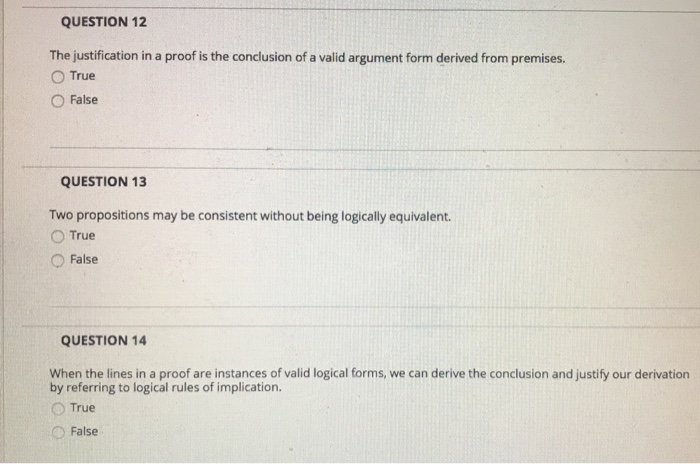QUESTION 12 The justification in a proof is the conclusion of a valid argument form derived from premises O True O False QUESTION 13 Two propositions may be consistent without being logically equivalent. O True O False QUESTION 14 When the lines in a proof are instances of valid logical forms, we can derive the conclusion and justify our derivation by referring to logical rules of implication. O True False QUESTION 44 What is the conclusion of the following syllogism?...

• ### 1. You have two independent samples, Xi,..., Xn and Yi,... , Ym drawn from populations with...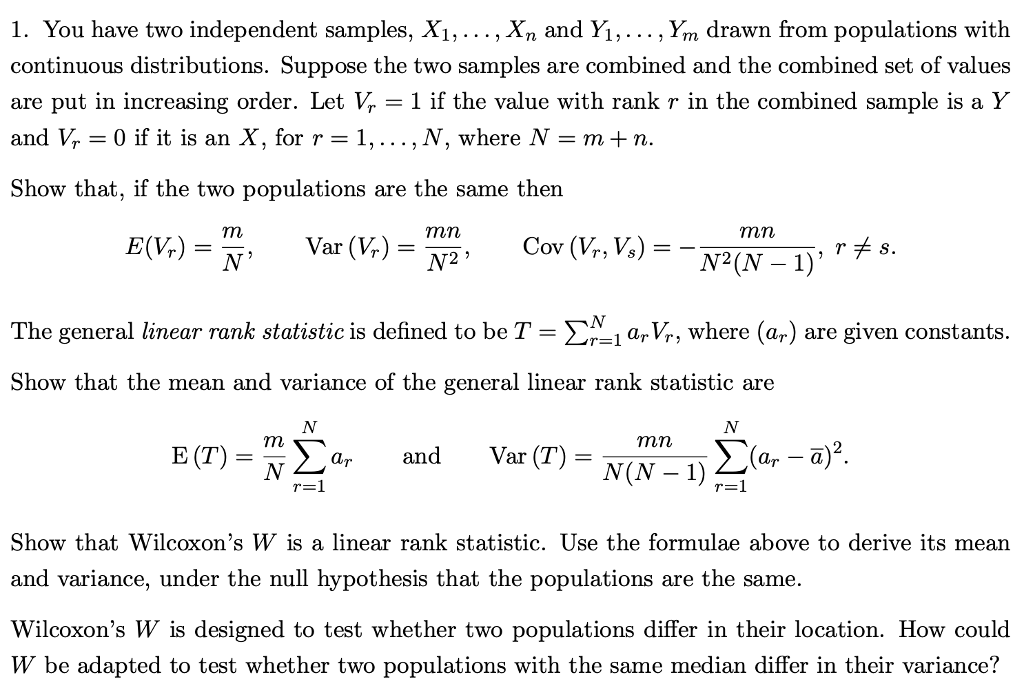1. You have two independent samples, Xi,..., Xn and Yi,... , Ym drawn from populations with continuous distributions. Suppose the two samples are combined and the combined set of values are put in increasing order. Let Vr- 1 if the value with rank r in the combined sample is a Y and V,-0 if it is an X, for r-1, . . . ,N, where N-m+ n. Show that, if the two populations are the same then mn E(V) TES...

• ### Exercise 1: The Taylor series for In(y) about y = 1 is (4) In(y) = 9...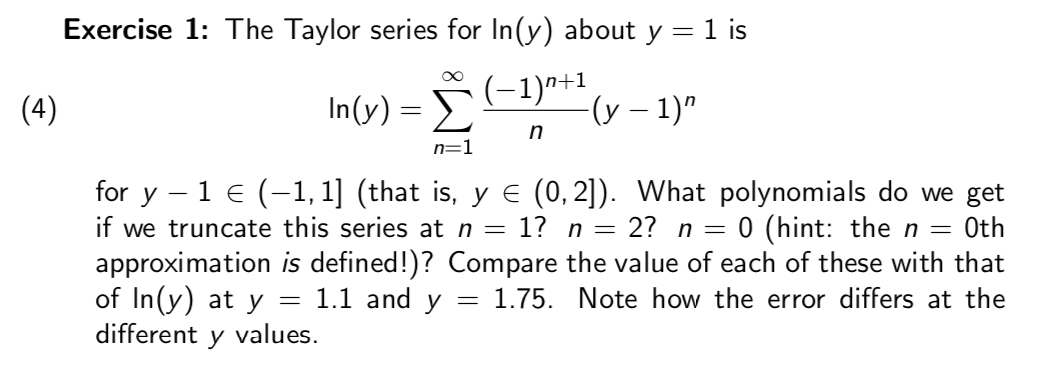Exercise 1: The Taylor series for In(y) about y = 1 is (4) In(y) = 9 (-1)"+(v - 1) n=1 for y-1€ (-1,1] (that is, y E (0,2]). What polynomials do we get if we truncate this series at n = 1? n = 2? n = 0 (hint: the n = Oth approximation is defined!)? Compare the value of each of these with that of In(y) at y = 1.1 and y = 1.75. Note how the error differs...

• ### 1 Semiquantitative Results Using Semiclassical Quantization In this problem, you will analyze the consequences of the...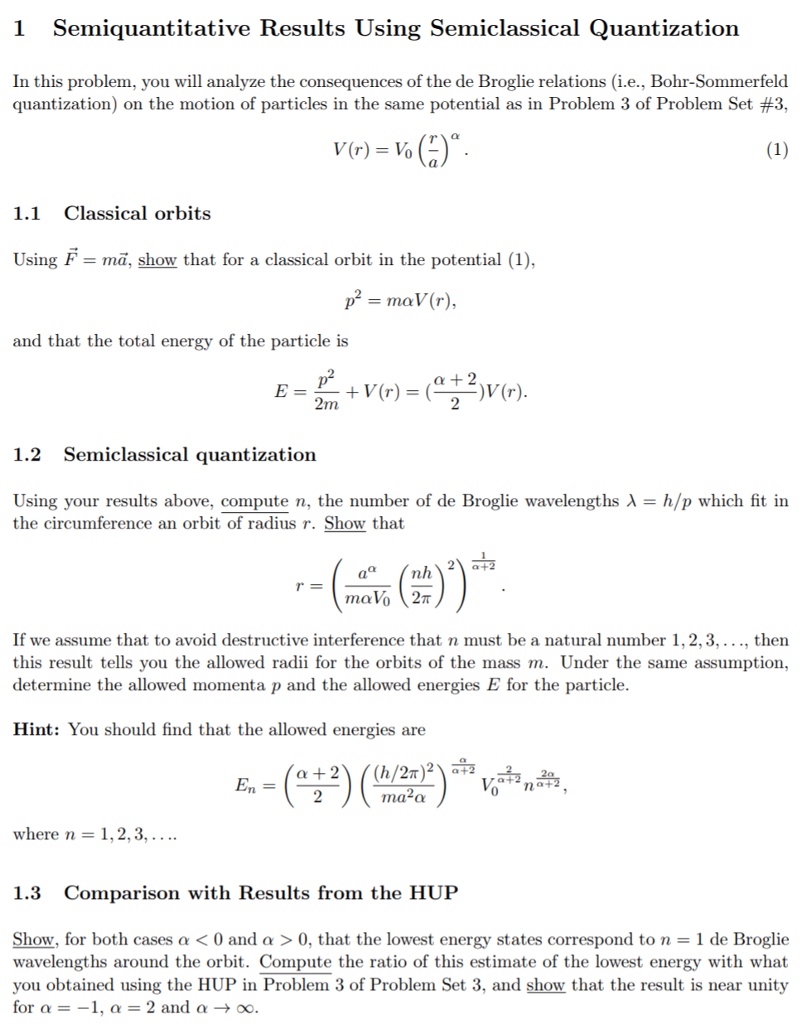1 Semiquantitative Results Using Semiclassical Quantization In this problem, you will analyze the consequences of the de Broglie relations (i.e., Bohr-Sommerfeld quantization) on the motion of particles in the same potential as in Problem 3 of Problem Set #3, V(r) = v. ()°. (1) 1.1 Classical orbits Using F = mã, show that for a classical orbit in the potential (1), pº = mav (r), and that the total energy of the particle is E = + V(r) = (;?(r)....

• ### Please do exercise 129: Exercise 128: Define r:N + N by r(n) = next(next(n)). Let f:N...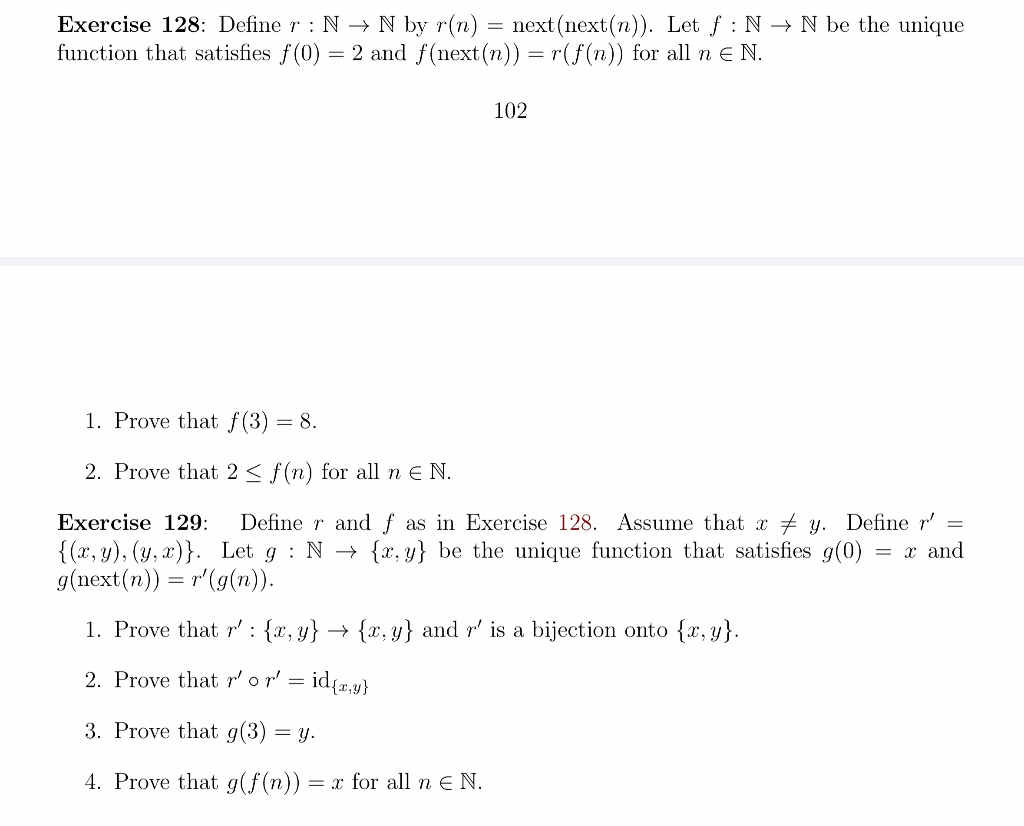Please do exercise 129: Exercise 128: Define r:N + N by r(n) = next(next(n)). Let f:N → N be the unique function that satisfies f(0) = 2 and f(next(n)) =r(f(n)) for all n E N. 102 1. Prove that f(3) = 8. 2. Prove that 2 <f(n) for all n E N. Exercise 129: Define r and f as in Exercise 128. Assume that x + y. Define r' = {(x,y),(y,x)}. Let g:N + {x,y} be the unique function that...

• ### Problem 05 In R Out For the circuit above, answer the following questions. 1. Derive the formula ...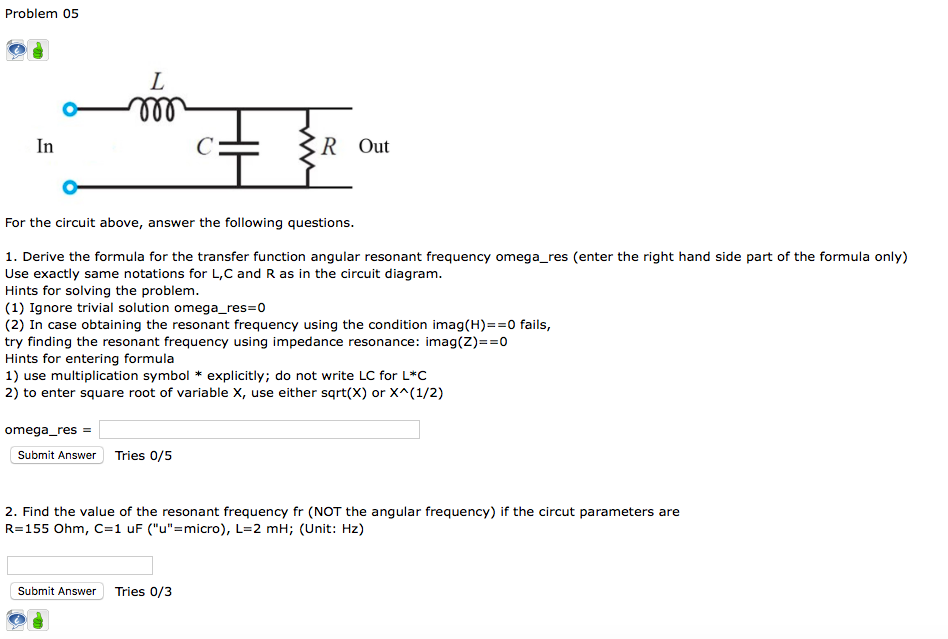Please Help! Problem 05 In R Out For the circuit above, answer the following questions. 1. Derive the formula for the transfer function angular resonant frequency omega_res (enter the right hand side part of the formula only) Use exactly same notations for L,C and R as in the circuit diagram. Hints for solving the problem. (1) Ignore trivial solution omega_res 0 (2) In case obtaining the resonant frequency using the condition imag(H)0 fails, try finding the resonant frequency using impedance...

• ### could you please solve a and b? Chapier 2i. Note: you needn't derive Kepler's laws-but do...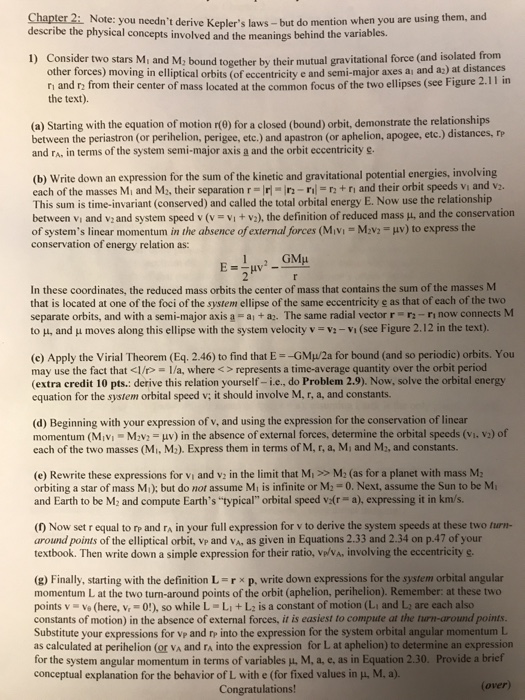could you please solve a and b? Chapier 2i. Note: you needn't derive Kepler's laws-but do mention when you are using them, an describe the physical concepts involved and the meanings behind the variables. u) Consider two stars Mi and M; bound together by their mutual gravitational force (and isolated from other forces) moving in elliptical orbits (of eccentricity e and semi-major axes ai and az) at distances 11 in n and r from their center of mass located at...

• ### Program Overview This brief exercise is designed for you to consider how reference variables behave when...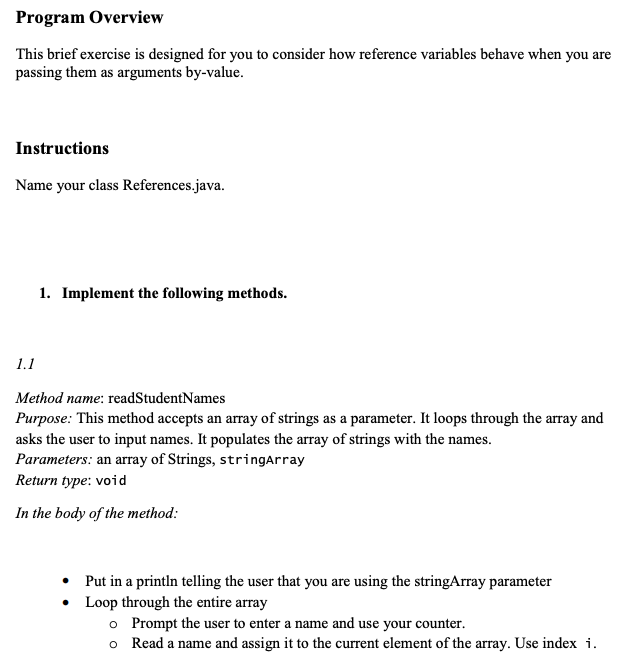Program Overview This brief exercise is designed for you to consider how reference variables behave when you are passing them as arguments by-value. Instructions Name your class References.java. 1. Implement the following methods. 1.1 Method name: readStudentNames Purpose: This method accepts an array of strings as a parameter. It loops through the array and asks the user to input names. It populates the array of strings with the names. Parameters: an array of Strings, stringArray Return type: void In the...

• ### Multivariable Calculus help with the magnitude of angular momentum: My questions is exercise 4 but I have attached exercise 1 and other notes that I was provided 4 Exercise 4. In any mechanics proble...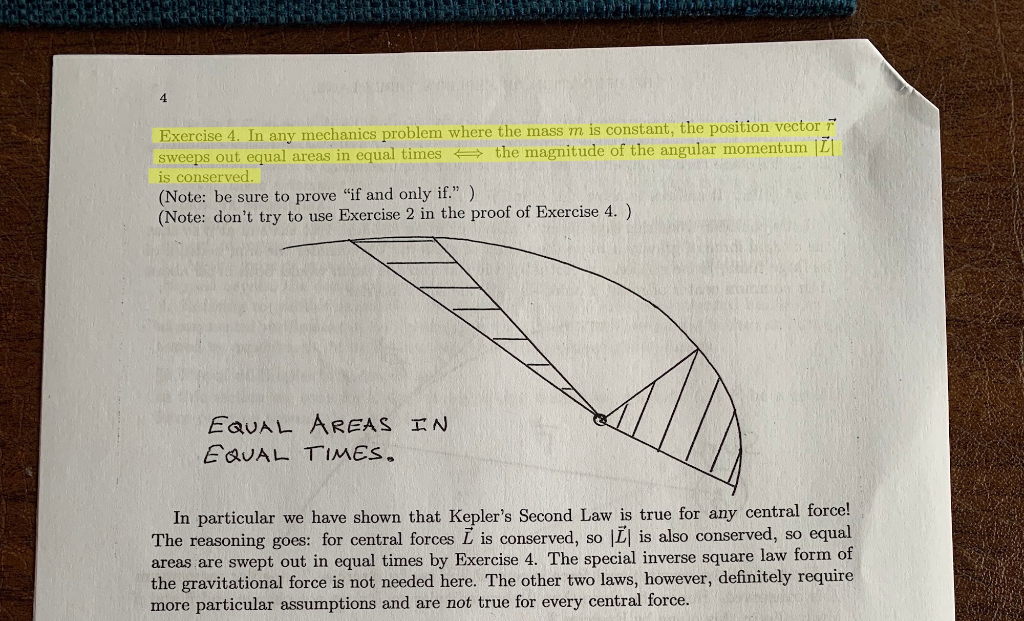Multivariable Calculus help with the magnitude of angular momentum: My questions is exercise 4 but I have attached exercise 1 and other notes that I was provided 4 Exercise 4. In any mechanics problem where the mass m is constant, the position vector F sweeps out equal areas in equal times the magnitude of the angular momentum ILI is conserved (Note: be sure to prove "if and only if") (Note: don't try to use Exercise 2 in the proof of...

• ### 1. Teresa Drops a Stone. (Allotted Time - 40 minutes) Teresa drops a stone from rest...

1. Teresa Drops a Stone. (Allotted Time - 40 minutes) Teresa drops a stone from rest from the top of an extremely tall building on a huge planet with essentially no atmosphere. You may assume that the stone experiences a constant gravitational acceleration as it falls to the planet’s surface, but the magnitude of this acceleration may not be equal to that on Earth. Let d1 be the distance the rock travels during the 1st second of its fall, let...